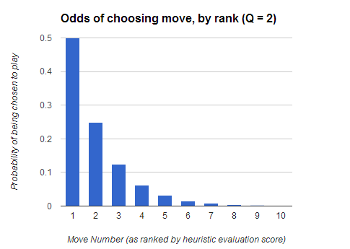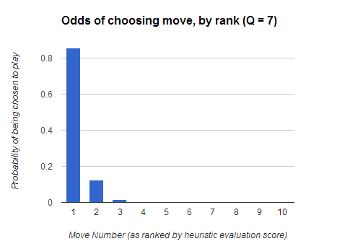# Adding some randomness to turn-based AI (2 of 2)

We just released a big update to Hive which included some great visual changes as well as a number of AI improvements. One of the interesting AI changes was the addition of a bit more randomness to the AI’s decisions, with minimal impact on the skill of the AI. This is the 2nd part in a 2-post series on the topic. If you haven’t read the first post of the series, I recommend that you at least skim it first.

## Randomness on all moves

Even with a large number of random openings, it would be possible to run into similar situations down the road that would play out time and time again (Battlestar Galactica style). Since this repetition would lead to predictability but most randomness decreases the skill-level of the AI, we had to strike a balance. In order to make it so that full games diverge from each other significantly, but without weakening the AI too much, I came up with the concept of a “randomness quotient”. I don’t know if this already exists in Game AI or not, but it seems to be a good solution and that was the most appropriate name I could think of for it.

## “Randomness Quotient” explained

The “randomness quotient” is a setting that will increase the randomness of the choices as its value decreases. Specifically, if the randomness quotient (which we will represent with the variable “Q”) is 7, then the engine will choose a ply other than the best move about 1/7 times (which is “1/Q” times). Of those instances where the best-scored ply is not chosen, then the second-best move will be chosen 1/Q times. Therefore, the odds of getting to the second best move and still choosing to consider the third-best scored move is ((1 / Q) / Q) which is the same as (1 / (Q ^ [move-number])). To figure out the opposite number (the odds of choosing move number N, rather than the odds that we’ll skip past it) we’d use a numerator of (Q-1). For example, the 8th best move would be chosen once in approximately ((Q-1) / (Q ^ 8)) moves. The more general equation is: ((Q-1) / (Q^N)) where Q is the randomness quotient, and N is the move number (as ranked by the heuristic evaluation method so that move 1 scores the best, move 2 scored the second best, etc.).

Even with a fairly high amount of randomness – such as a Q of 2, remember: low Q means high randomness – we would only see a move as bad as the 8th move in 1 out of every (1/(2^8))= 256 moves.

These occasional less-than-stellar moves are essentially simulating a human player occasionally losing concentration.

In case the math was a bit confusing there, we can show the effects of this algorithm visually. Each bar in the chart is the move-number where the moves are ranked from the best-scoring on the left to the worst-scoring on the right.In the first chart, there is a very low randomness quotient of “2” which is NOT recommended in realistic play, but it’s still a great example to visualize how this works. You’ll notice the first move is chosen 1/2 of the time. Since the other 1/2 of the moves are also cut in half, then the second-best move (move index 1) is only chosen in 1/4 of the instances.In this second chart, we have a higher randomness quotient of 7 which represents a more realistic setting. This setting doesn’t add a ton of randomness to any given move, but over the length of a normal game, this should introduce enough randomness to steer any similar games apart from each other. As you may have recognized, these charts are an example of exponential decay.

In our code, we used lower Q values to give more randomness to weaker levels of AI to “nerf” them a bit, while giving them more variation at the same time. The higher levels of AI still have a modestly high randomness quotient since we want a little randomness – currently Q is around 8 or 9 – but we may continue to tweak that from experience.

## Randomness Quotient pseudocode

This is almost the exact code from our Minimax engine where we implement randomness based on the Randomness Quotient. It’s not that much code!

```// At this point, the Minimax engine has scored some plies
// and stored them and their scores in topLevelScoresByPly.
List<KeyValuePair<Ply, double>> plyPairList = topLevelScoresByPly.ToList();

// Sort the plies descending by their scores (best score at index 0).
plyPairList.Sort((x, y) => y.Value.CompareTo(x.Value));

// Apply the RandomnessQuotient so that the best-scored ply is not guaranteed to be chosen, but is still
// exponentially more likely than the second which is exponentially more likely than the third, etc.
int plyIndex = 0;
while ((plyIndex < (plyPairList.Count - 1)) && (0 == randGen.Next((int)RandomnessQuotient)))
{
plyIndex++;
}
if (plyIndex > 0)
{
Logger.log(LogLevel.INFO, "RANDOMNESS! Skipping the best (0th) ply and chosing ply index " + plyIndex +" instead.");
}

// Grab the ply that the Randomness Quotient has chosen.
theChosenPly = plyPairList[plyIndex].Key;
chosenPlyScore = plyPairList[plyIndex].Value;```

## Conclusion

The addition of randomness to the AI engine has greatly increased the replay-value of the AI in Hive. Hopefully this concept can be useful to some other developers as well. This change was released as a free update two days ago, along with a significant batch of visual updates and about a half-dozen other AI improvements that we’ve made over the past week or so. If you have the game, check them out… if you haven’t bought Hive yet, please support us by grabbing your copy on Steam today!